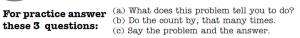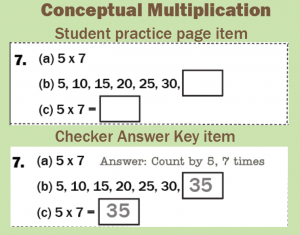# Conceptual Multiplication (count-bys)

### Conceptual Multiplication teaches the concept of multiplication. Who needs it?

Students who have not been introduced to the concept of multiplication–typically 2nd graders or early 3rd grades need conceptual multiplication.  It is based upon skip counting or count-bys.  In this Learning Track students can read all of the count-by series except the last number, making prior mastery of the skip counting sequences unnecessary.

Students in fourth grade and above (we are in a hurry, as they are late) should be able to do this Learning Track without doing Skip Counting first.   Students do NOT need this learning track at all if they are able to figure out the answer to any multiplication fact when you ask them, for example, “What’s seven times eight?”  If they can figure out the answer to that question, then they already understand the concept of multiplication.

Also, students in fourth grade and above (we’re still in a hurry!) do not need to finish all the way to level Z.  They probably have the concept by the time they finish Set H and can go on into the Multiplication (1s-9s) Learning Track.  Just sayin’ we’re in a hurry!

## How does it teach?

See this video on how a pair should practice.In each box there are 3 questions: a, b, and c. The checker will only need to read these questions a few times until the student knows what is expected at each letter.(a)  What does this problem tell you to do? (ex: 5 x 7)    Answer: Count by five, seven times.

(b)  Do the count by, that many times. Answer: 5, 10, 15, 20, 25, 30, 35.

(c) Say the problem and the answer. Answer: Five times seven equals 35.

After a time, the student should just say the answers, for example, “Count by 5 seven times. 5, 10, 15, 20, 25, 30, 35.  Five times seven equals 35.”

For the One-Minute Test students just write the answers in the boxes. In the above example the student would write “35” in both boxes.